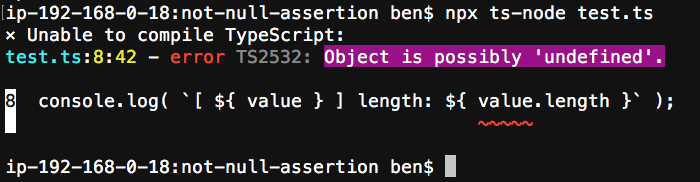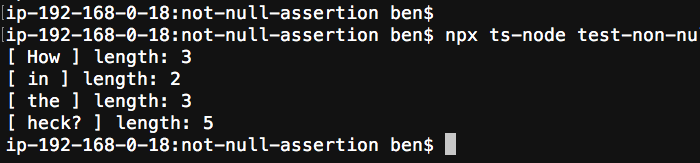# Using The Non-Null Assertion Operator To Fix .shift() and .pop() Errors In TypeScript 3

By on

One of the things that I love most about TypeScript is that it forces me to think more deeply about my code because it is constantly checking the "assumptions" that I make about runtime truths. Sometimes, however, TypeScript can't deduce things that I know to be true. For example, when working with Arrays, TypeScript believes that the Array methods .shift() and .pop() may return "undefined" regardless of the context in which they are being called. In such cases, we can use the "Non-Null Assertion" operator (!) to tell TypeScript that a given expression will always evaluate to a non-null value.

NOTE: This looks like, but should not be confused with, the "Definite Assignment Assertion" in TypeScript. Though, if you squint hard enough, the two uses of (!) do share somewhat similar intents.

First, let's put together a simple example of TypeScript failing to know that a value will be defined:

``````var things: string[] = [ "How", "in", "the", "heck?" ];

while ( things.length ) {

var value = things.shift();

console.log( `[ \${ value } ] length: \${ value.length }` );

}
``````

In this case, we know that "things" is a collection that only contains strings. And, we know that the body of our while-loop will only be evaluated when there are values remaining in the collection. And, we know that we are only calling .shift() once per iteration. As such, we can deduce that the .shift() call will always return a defined value. However, when we run this code, TypeScript throws the following error:

Object is possibly 'undefined'.This comes up a lot in my Angular code (which is written in TypeScript). And, for a long time, I've been getting around this by explicitly casting the resultant value to a what I know will be the returned type:

``````var things: string[] = [ "How", "in", "the", "heck?" ];

while ( things.length ) {

// At this point, we know that "things" has values in it (since the while-loop hasn't
// been terminated). And, we know that this collection only contains strings. As
// such, we know that the shifted "value" will ALWAYS BE A STRING at this point in
// the code. We can, therefore, cast the returned value to the appropriate type.
var value = ( things.shift() as "string" );

console.log( `[ \${ value } ] length: \${ value.length }` );

}
``````

This works. But, it's wordy and it's unfortunate that I need to hard-code the Type into the code. I'd much rather let TypeScript use type-inference.

Luckily, I recently came across the "Non-Null Assertion" operator (!) in TypeScript which will tell the TypeScript compiler that the value returned by the .shift() call will always be non-null.

``````var things: string[] = [ "How", "in", "the", "heck?" ];

while ( things.length ) {

// At this point, we know that "things" has values in it (since the while-loop hasn't
// been terminated). And, we know that this collection only contains strings. As
// such, we know that the shifted "value" will ALWAYS BE NON-NULL at this point in
// the code. As such, we can use the "non-null assertion operator" to tell TypeScript
// to relax the null check on the evaluated expression.
var value = things.shift() !;

console.log( `[ \${ value } ] length: \${ value.length }` );

}
``````

As you can see, we've placed the non-null assertion operator (!) after the .shift() call. This tells TypeScript that the expression preceding the "!" operator will be non-null. TypeScript will therefore assume that the value returned by .shift() is of type "string" since that is how we've typed the Array.

And, when we run this through ts-node / TypeScript, we get the following terminal output:To be clear, this doesn't actually change the emitted code at all. So, if the value is really undefined at runtime, we'll still get a runtime error. The non-null assertion operator is just a way for us to tell TypeScript about the assumptions that can be made at compile-time.

For the most part, TypeScript is great at deducing the Type of a value at compile time, especially when using Type Guards. But sometimes, even a guard condition isn't enough to convince the TypeScript compiler that a value will be non-null. In such cases, we can use the non-null assertion operator (!) to bridge the gap while keeping the code short and easy to read.

Want to use code from this post? Check out the license.